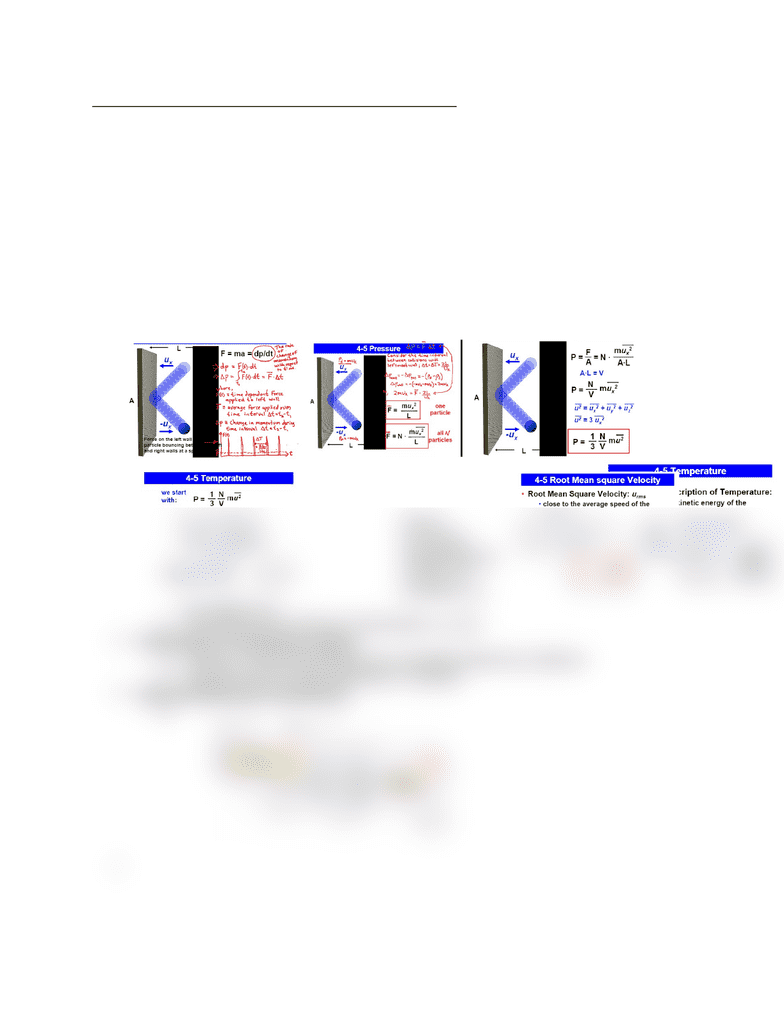Class Notes (1,000,000)
CA (610,000)
McGill (30,000)
Chemistry (1,000)
CHEM 120 (100)
Lecture 4

CHEM 120 Lecture Notes - Lecture 4: Molar Mass, Histogram

Department
Chemistry
Course Code
CHEM 120
Professor
Lecture
4

This preview shows half of the first page. to view the full 2 pages of the document.CHEM 120 – Lecture 4 – Molecular kinetic theory of gases
Behaviour of ideal gases can be explained by a simple model
oLarge number of particles all following newton’s laws of motion
Large number of particles have random, straight line motion, separated by large
distances
Collisions between them are brief though there is no long-range forces between particles
Gas has internal energy due to kinetic energy of particles; total energy is thus constant
Microscopic description of pressure
oCollisions of particles with walls of container
oP=Nmu2/3V, u is average square velocity, N number of particles, m is mass of
particle
Derivation
Root mean square
velocity
oIf the numerator
has an
increasing
temperature by
a factor of 4
increases urms
by a factor of 2
oIncreasing molar mass by 4 decreases urms by 2
Distribution of molecular speeds
oVelocity of individual particles are constantly altered by collisions
oParticles haves a wide distribution of speeds
Maxwell-Boltzmann distribution
o